Skip to main content

# Positive explicit and implicit computational techniques for reaction–diffusion epidemic model of dengue disease dynamics

## Abstract

The aim of this work is to develop a novel explicit unconditionally positivity preserving finite difference (FD) scheme and an implicit positive FD scheme for the numerical solution of dengue epidemic reaction–diffusion model with incubation period of virus. The proposed schemes are unconditionally stable and preserve all the essential properties of the solution of the dengue reaction diffusion model. This proposed FD schemes are unconditionally dynamically consistent with positivity property and converge to the true equilibrium points of dengue epidemic reaction diffusion system. Comparison of the proposed scheme with the well-known existing techniques is also presented. The time efficiency of both the proposed schemes is also compared, with the two widely used techniques.

## Introduction

Dengue fever is a mosquito born infection which causes flu-like illness, fever and severe pain in the body. Dengue virus is transmitted by Aedes mosquito bite. It is a lethal disease that starts with painful fever. Some people have non-febrile illness with rash, headache, pain behind eyes and joint pains. Dengue hemorrhagic fever is highly complicated which can cause high fever, hemorrhage and enlargement of liver and circulatory failure. Dengue is an epidemic disease which can be prevented by awareness programs against it. There is no vaccine and specific medication to treat it. In order to get a good understanding of the nature and dynamics of the transmission of dengue epidemics, various epidemic models of dengue disease dynamics are discussed in the literature . Most of the epidemic models of infection disease dynamics are based on ODE systems. Recently, the researchers were developing integer order models in the setup of fractional calculus. Since the fractional calculus is an extension of the classical calculus, its scope is wider than that of its counterpart. Epidemic models, in the framework of fractional calculus, address more parameters which reduces errors. Also, by including these parameters, the models express a very close behavior to the actual physical problem. Several authors used various fractional operators for developing epidemic models, for detail, see the references . These models provide a motivation to the young researchers.

In order to get appropriate perception of dispersal and control of dengue transmission, it is important to consider the dengue epidemic model with diffusion because individuals do not mix homogenously.

Let us consider a dengue epidemic model proposed by Rafiq et al. :

\begin{aligned}& \frac{dS}{dt} = \mu _{h} - \beta _{h} {S} I_{v} ({C}/ \mu _{v} )- \mu _{h} S, \end{aligned}
(1.1)
\begin{aligned}& \frac{dX}{dt} =-( \alpha _{h} + \mu _{h} )X+ \beta _{h} {S} I_{v} ({C}/ \mu _{v} ), \end{aligned}
(1.2)
\begin{aligned}& \frac{dI}{dt} =- ( r + \mu _{h} ) I+ \alpha _{h} X, \end{aligned}
(1.3)
\begin{aligned}& \frac{d X_{v}}{dt} =- ( \alpha _{v} + \mu _{v} ) X_{v} + \beta _{v} I N_{T} ( 1- X_{v} - I_{v} ), \end{aligned}
(1.4)
\begin{aligned}& \frac{d I_{v}}{dt} =- \mu _{v} I_{v} + \alpha _{v} X_{v}, \end{aligned}
(1.5)

and the conditions

$$S+X+I+R=1\quad \text{and} \quad S_{v} + X_{v} + I_{v} =1.$$
(1.6)

Note that (1.1)–(1.5) is a normalized system discussed by M. Rafiq et al. .

Let $$d_{S}$$, $$d_{X}$$, $$d_{I}$$, $$d_{X_{v}}$$ and $$d_{I_{v}}$$ be the diffusive constants of S, X, I, $$X_{v}$$ and $$I_{v}$$, respectively. Then system (1.1)–(1.5) with diffusion can be written as

\begin{aligned}& \frac{\partial S}{\partial t} = d_{S} \frac{\partial ^{2} S}{\partial x^{2}} + \mu _{h} - \beta _{h} {S} I_{v} ({C}/ \mu _{v} )- \mu _{h} S, \end{aligned}
(1.7)
\begin{aligned}& \frac{\partial X}{\partial t} = d_{X} \frac{\partial ^{2} X}{\partial x^{2}} + \beta _{h} {S} I_{v} ({C}/ \mu _{v} )- \alpha _{h} X - \mu _{h} X, \end{aligned}
(1.8)
\begin{aligned}& \frac{\partial I}{\partial t} = d_{I} \frac{\partial ^{2} I}{\partial x^{2}} + \alpha _{h} X - rI - \mu _{h} I, \end{aligned}
(1.9)
\begin{aligned}& \frac{\partial X_{v}}{\partial t} = d_{X_{v}} \frac{\partial ^{2} X_{v}}{\partial x^{2}} + \beta _{v} I N_{T} (1- X_{v} - I_{v} )- \alpha _{v} X_{v} - \mu _{v} X_{v}, \end{aligned}
(1.10)
\begin{aligned}& \frac{\partial I_{v}}{\partial t} = d_{I_{v}} \frac{\partial ^{2} I_{v}}{\partial x^{2}} + \alpha _{v} X_{v} - \mu _{v} I_{v}, \end{aligned}
(1.11)

where $$S ( x,t )$$, $$X ( x,t )$$ and $$I ( x,t )$$ are population sizes of susceptible, exposed, and infectious humans, while $$X_{v} ( x,t )$$ and $$I_{v} ( x,t )$$ are population sizes of exposed and infectious vectors, respectively, at location x and time t, where $$\mu _{h}$$, $$\beta _{h}$$, $$\alpha _{h}$$, r and $$N_{T}$$ are the rate of death of humans population, infection rate from vector population to human population, rate at which infected human population becomes infectious, recovery rate for human population and total human population, respectively. The rates $$\beta _{v}$$, $$\alpha _{v}$$, $$\mu _{v}$$ and C are infection rate from human population to vector population, rate at which infected vector population becomes infectious, death rate of vector population and recruitment rate of vector population, respectively.

The main theme of this work is to provide numerical techniques which are consistent to the continuous diffusive epidemic model. The epidemic models describe the unknown quantities as population sizes therefore negative solutions of epidemic models are meaningless. In order to find the solution of dengue epidemic model, the method of solution should preserve the positivity of the solution. Also proposed epidemic models of dengue dynamics have two stable equilibrium points, the numerical technique must show convergence towards these equilibrium points. Normally, classical and well-known numerical techniques have flaws in their construction, therefore these techniques cannot preserve most of the properties possessed by the continuous epidemic models. The proposed techniques not only preserve the positive solution but also converge towards the true equilibrium points of the continuous system.

## Equilibrium points

Dengue epidemic model (1.7)–(1.11) describes two possible equilibrium points, disease free equilibrium (DFE) and endemic equilibrium (EE), namely

• DFE

$$E_{0} (1, 0, 0,0,0),$$
• EE

$$E_{1} \bigl( S^{*}, X^{*}, I^{*}, X_{v}^{*}, I_{v}^{*} \bigr),$$

where

\begin{aligned}& S^{*} = \frac{ ( \alpha _{v} + \mu _{v} ) (MN \mu _{h}^{2} \mu _{v} + \alpha _{h} \gamma _{v} \mu _{h} )}{\alpha _{h} \gamma _{v} [ \mu _{h} ( \alpha _{v} + \mu _{v} ) + \alpha _{v} \gamma _{h} ]}, \\& X^{*} = \frac{M \mu _{h}^{2} \mu _{v} ( \alpha _{v} + \mu _{v} ) ( \mathfrak{R}_{0} - 1)}{\alpha _{h} \alpha _{h} [ \mu _{h} ( \alpha _{v} + \mu _{v} ) +\alpha _{v} \gamma _{h} ]}, \\& I^{*} = \frac{\mu _{h} \mu _{v} ( \alpha _{v} + \mu _{v} ) ( \mathfrak{R}_{0} - 1)}{\alpha _{h} [ \mu _{h} ( \alpha _{v} + \mu _{v} ) +\alpha _{v} \gamma _{h} ]}, \\& X_{v}^{*} = \frac{\mu _{v} (MN \mu _{h}^{3} \mu _{v} ) ( \mathfrak{R}_{0} - 1)}{\gamma _{h} \alpha _{v} ( \alpha _{h} \gamma _{v} \mu _{h} +MN \mu _{h}^{2} \mu _{v} )}, \\& I_{v}^{*} = \frac{MN \mu _{h}^{3} \mu _{v}}{\gamma _{h} ( \alpha _{h} \gamma _{v} \mu _{h} +MN \mu _{h}^{2} \mu _{v} )} ( \mathfrak{R}_{0} - 1 ), \end{aligned}

and where

$$\mathfrak{R}_{0} = \frac{\alpha _{h} \alpha _{v} \gamma _{h} \gamma _{v}}{ ( r+ \mu _{h} ) ( \alpha _{h} + \mu _{h} ) \mu _{v} ( \alpha _{v} + \mu _{v} )},\quad \text{when } d_{S} = d_{X} = d_{I} = d_{X_{v}} = d_{I_{v}} =0,$$

is the reproductive number.

Also

$$\gamma _{h} = \beta _{h} \biggl( \frac{C}{\mu _{v}} \biggr),\qquad \gamma _{v} = \beta _{v} N_{T}, \qquad M= \frac{r+ \mu _{h}}{\mu _{h}}\quad \text{and} \quad N= \frac{\alpha _{h} + \mu _{h}}{\mu _{h}}.$$

The reproductive number $$\mathfrak{R}_{0}$$ decides the outcome, namely if $$\mathfrak{R}_{0} <1$$, the disease is eliminated from the given population, and if $$\mathfrak{R}_{0} >1$$, the disease persists in the population.

## Computational method

A nonstandard FD method, introduced by Mickens , is an efficient tool to solve epidemic models because this method is structure-preserving and consistent with the solution of epidemic models. Many researchers used structure-preserving methods to solve epidemic models with a system of ordinary and partial differential equations [11, 1322]. For the reaction–diffusion epidemic model and some of the work concerning positivity-preserving finite difference schemes, we refer to the literature .

In the present study, we design two structure-preserving finite difference schemes  for dengue epidemic model with diffusion. The proposed schemes are convergent towards all steady states of the continuous system and preserve positivity property that highlights the significance and efficacy of the proposed schemes.

In this section, we rewrite system (1.7)–(1.11) as

\begin{aligned}& \frac{\partial S}{\partial t} = d_{S} \frac{\partial ^{2} S}{\partial x^{2}} + \mu _{h} - \beta _{h} {S} I_{v} ({C}/ \mu _{v} )- \mu _{h} S, \end{aligned}
(3.1)
\begin{aligned}& \frac{\partial X}{\partial t} = d_{X} \frac{\partial ^{2} X}{\partial x^{2}} + \beta _{h} {S} I_{v} ({C}/ \mu _{v} )- \alpha _{h} X - \mu _{h} X, \end{aligned}
(3.2)
\begin{aligned}& \frac{\partial I}{\partial t} = d_{I} \frac{\partial ^{2} I}{\partial x^{2}} + \alpha _{h} X - rI - \mu _{h} I, \end{aligned}
(3.3)
\begin{aligned}& \frac{\partial X_{v}}{\partial t} = d_{X_{v}} \frac{\partial ^{2} X_{v}}{\partial x^{2}} + \beta _{v} I N_{T} (1- X_{v} - I_{v} )- \alpha _{v} X_{v} - \mu _{v} X_{v}, \end{aligned}
(3.4)
\begin{aligned}& \frac{\partial I_{v}}{\partial t} = d_{X_{v}} \frac{\partial ^{2} I_{v}}{\partial x^{2}} + \alpha _{v} X_{v} - \mu _{v} I_{v}, \end{aligned}
(3.5)

for all $$t\geq 0$$, $$x\in [ 0,L ]$$ and the initial conditions are:

\begin{aligned}& S ( x,0 ) = \delta _{1} ( x ) \geq 0,\quad\quad X ( x,0 ) = \delta _{2} ( x ) \geq 0, \quad\quad I ( x,0 ) = \delta _{3} ( x ) \geq 0, \end{aligned}
(3.6)
\begin{aligned}& X_{v} (x,0)= \delta _{4} ( x ) \geq 0\quad \text{and} \quad I_{v} (x,0)= \delta _{5} ( x ) \geq 0, \end{aligned}
(3.7)

while the boundary conditions are:

\begin{aligned}& \frac{\partial S(0,t)}{\partial x} = \frac{\partial X(0,t)}{\partial x} = \frac{\partial I(0,t)}{\partial x} = \frac{\partial X_{v} (0,t)}{\partial x} = \frac{\partial I_{v} (0,t)}{\partial x} =0,\quad t>0, \end{aligned}
(3.8)
\begin{aligned}& \frac{\partial S(L,t)}{\partial x} = \frac{\partial X(L,t)}{\partial x} = \frac{\partial I(L,t)}{\partial x} = \frac{\partial X_{v} (L,t)}{\partial x} = \frac{\partial I_{v} (L,t)}{\partial x} =0, \quad t>0. \end{aligned}
(3.9)

Divide $$[ 0,L ] \times [ 0,T ]$$ using $$M\times N$$ mesh points having time and space step sizes $$h= \frac{L}{M}$$ and $$\tau = \frac{T}{N}$$.

The nodal points then are

\begin{aligned}& x_{i} =ih, \quad i=0,1,2,\ldots,M, \\& t_{n} =n\tau , \quad n=0,1,2,\ldots,N, \end{aligned}

$$S_{i}^{n}$$, $$X_{i}^{n}$$, $$I_{i}^{n}$$, $$X_{v i}^{n}$$ and $$I_{v i}^{n}$$ are denoted as FD values of $$S ( ih,n\tau )$$, $$X ( ih,n\tau )$$, $$I(ih,n\tau )$$, $$X_{v} (ih,n\tau )$$ and $$I_{v} ( ih,n\tau )$$, respectively.

Four FD schemes are used to solve system (3.1)–(3.5) numerically: forward Euler explicit FD scheme, Crank–Nicolson implicit FD scheme and the proposed FD scheme. Forward Euler FD scheme for system (3.1)–(3.5) is:

\begin{aligned}& S_{i}^{n+1} = S_{i}^{n} + R_{1} \bigl( S_{i-1}^{n} -2 S_{i}^{n} + S_{i+1}^{n} \bigr) +\tau \mu _{h} -\tau \beta _{h} (C/ \mu _{h} ) S_{i}^{n} I_{v i}^{n} -\tau \mu _{h} S_{i}^{n}, \\& X_{i}^{n+1} = X_{i}^{n} + R_{2} \bigl( X_{i-1}^{n} -2 X_{i}^{n} + X_{i+1}^{n} \bigr) +\tau \beta _{h} S_{i}^{n} I_{v i}^{n} \biggl( \frac{C}{\mu _{h}} \biggr) -\tau ( \mu _{h} + \alpha _{h} ) X_{i}^{n}, \\& I_{i}^{n+1} = I_{i}^{n} + R_{3} \bigl( I_{i-1}^{n} -2 I_{i}^{n} + I_{i+1}^{n} \bigr) +\tau \alpha _{h} X_{i}^{n} -\tau (r+ \mu _{h} ) I_{i}^{n}, \\& X_{v i}^{n+1} = X_{v i}^{n} + R_{4} \bigl( X_{v i-1}^{n} -2 X_{v i}^{n} + X_{v i+1}^{n} \bigr) +\tau \beta _{v} N_{t} I_{i}^{n} \bigl( 1- X_{v i}^{n} - I_{v i}^{n} \bigr) -\tau (\alpha _{v} + \mu _{v} ) X_{v i}^{n}, \\& I_{v i}^{n+1} = I_{v i}^{n} + R_{5} \bigl( I_{v i-1}^{n} -2 I_{v i}^{n} + I_{v i+1}^{n} \bigr) + \tau \alpha _{v} X_{v i}^{n} - \tau \mu _{v} I_{v i}^{n}. \end{aligned}

The stability range of Forward Euler explicit scheme is $$R_{1} \leq \frac{2-\tau \mu _{h}}{4}$$, $$R_{2} \leq \frac{2-\tau ( \mu _{h} + \alpha _{h} )}{4}$$, $$R_{3} \leq \frac{2-\tau ( r+ \mu _{h} )}{4}$$, $$R_{4} \leq \frac{2-\tau (\alpha _{v} + \mu _{v} )}{4}$$ and $$R_{5} \leq \frac{2-\tau \mu _{v}}{4}$$.

Crank–Nicolson FD scheme for system (3.1)–(3.5) is:

\begin{aligned}& ( 1+ R_{1} ) S_{i}^{n+1} - \frac{R_{1}}{2} \bigl( S_{i-1}^{n+1} + S_{i+1}^{n+1} \bigr) \\& \quad = ( 1- R_{1} ) S_{i}^{n} + \frac{R_{1}}{2} \bigl( S_{i-1}^{n} + S_{i+1}^{n} \bigr)+\tau \mu _{h} -\tau \beta _{h} (C/ \mu _{h} ) S_{i}^{n} I_{v i}^{n} -\tau \mu _{h} S_{i}^{n}, \\& ( 1+ R_{2} ) X_{i}^{n+1} - \frac{R_{2}}{2} \bigl( X_{i-1}^{n+1} + X_{i+1}^{n+1} \bigr) \\& \quad= ( 1- R_{2} ) X_{i}^{n} + \frac{R_{2}}{2} \bigl( X_{i-1}^{n} + X_{i+1}^{n} \bigr)+\tau \beta _{h} S_{i}^{n} I_{v i}^{n} \biggl( \frac{C}{\mu _{h}} \biggr) -\tau ( \mu _{h} + \alpha _{h} ) X_{i}^{n}, \\& ( 1+ R_{3} ) I_{i}^{n+1} - \frac{R_{3}}{2} \bigl( I_{i-1}^{n+1} + I_{i+1}^{n+1} \bigr) = ( 1- R_{3} ) I_{i}^{n} + \frac{R_{3}}{2} \bigl( I_{i-1}^{n} + I_{i+1}^{n} \bigr)+\tau \alpha _{h} X_{i}^{n} -\tau (r+ \mu _{h} ) I_{i}^{n}, \\& ( 1+ R_{4} ) X_{v i}^{n+1} - \frac{R_{4}}{2} \bigl( X_{v i-1}^{n+1} + X_{v i+1}^{n+1} \bigr) \\& \quad= ( 1- R_{4} ) X_{v i}^{n} + \frac{R_{4}}{2} \bigl( X_{v i-1}^{n} + X_{v i+1}^{n} \bigr)+\tau \beta _{v} N_{t} I_{i}^{n} \bigl( 1- X_{v i}^{n} - I_{v i}^{n} \bigr) -\tau ( \alpha _{v} + \mu _{v} ) X_{v i}^{n}, \\& ( 1+ R_{5} ) I_{v i}^{n+1} - \frac{R_{5}}{2} \bigl( I_{v i-1}^{n+1} + I_{v i+1}^{n+1} \bigr) = ( 1- R_{5} ) I_{v i}^{n} + \frac{R_{5}}{2} \bigl( I_{v i-1}^{n} + I_{v i+1}^{n} \bigr) + \tau \alpha _{v} X_{v i}^{n} - \tau \mu _{v} I_{v i}^{n}. \end{aligned}

Crank–Nicolson scheme is unconditionally stable.

Now the proposed explicit positive FD scheme is developed  with the help of rules defined by Mickens  as follows:

\begin{aligned}& S_{i}^{n+1} = S_{i}^{n} + R_{1} \bigl( S_{i-1}^{n} + S_{i+1}^{n} \bigr) -2 R_{1} S_{i}^{n+1} +\tau \mu _{h} -\tau \beta _{h} I_{v i}^{n} (C/ \mu _{h} ) S_{i}^{n+1} -\tau \mu _{h} S_{i}^{n+1}, \end{aligned}
(3.10)
\begin{aligned}& S_{i}^{n+1} +\tau \beta _{h} I_{v i}^{n} \biggl( \frac{C}{\mu _{h}} \biggr) S_{i}^{n+1} +\tau \mu _{h} S_{i}^{n+1} +2 R_{1} S_{i}^{n+1} = S_{i}^{n} + R_{1} \bigl( S_{i-1}^{n} + S_{i+1}^{n} \bigr) +\tau \mu _{h}, \\& \biggl( 1+\tau \beta _{h} I_{v i}^{n} \biggl( \frac{C}{\mu _{h}} \biggr) +\tau \mu _{h} +2 R_{1} \biggr) S_{i}^{n+1} = S_{i}^{n} + R_{1} \bigl( S_{i-1}^{n} + S_{i+1}^{n} \bigr) +\tau \mu _{h}, \\& S_{i}^{n+1} = \frac{S_{i}^{n} + R_{1} ( S_{i-1}^{n} + S_{i+1}^{n} ) +\tau \mu _{h}}{1+\tau \beta _{h} I_{v i}^{n} ( \frac{C}{\mu _{h}} ) +\tau \mu _{h} +2 R_{1}}. \end{aligned}
(3.11)

A similar process is used and we get

\begin{aligned}& X_{i}^{n+1} = \frac{X_{i}^{n} + R_{2} ( X_{i-1}^{n} + X_{i+1}^{n} ) +\tau \beta _{h} S_{i}^{n} I_{v i}^{n} ( \frac{C}{\mu _{h}} )}{1+\tau \mu _{h} +\tau \alpha _{h} +2 R_{2}}, \end{aligned}
(3.12)
\begin{aligned}& I_{i}^{n+1} = \frac{I_{i}^{n} + R_{3} ( I_{i-1}^{n} + I_{i+1}^{n} ) +\tau \alpha _{h} X_{i}^{n}}{1+\tau r+\tau \mu _{h} +2 R_{3}}, \end{aligned}
(3.13)
\begin{aligned}& X_{v i}^{n+1} = \frac{X_{v i}^{n} + R_{4} ( X_{v i-1}^{n} + X_{v i+1}^{n} ) +\tau \beta _{v} N_{t} I_{i}^{n} (1- I_{v i}^{n} )}{1+\tau \beta _{v} N_{t} I_{i}^{n} +\tau \alpha _{v} +\tau \mu _{v} +2 R_{4}}, \end{aligned}
(3.14)
\begin{aligned}& I_{v i}^{n+1} = \frac{I_{v i}^{n} + R_{5} ( I_{v i-1}^{n} + I_{v i+1}^{n} ) + \tau \alpha _{v} X_{v i}^{n}}{1+\tau \mu _{v} +2 R_{5}}. \end{aligned}
(3.15)

Now we design the proposed positive implicit scheme for the given model (3.1)–(3.5) as:

\begin{aligned}& ( 1+ 2R_{1} ) S_{i}^{n+1} - R_{1} \bigl( S_{i-1}^{n+1} + S_{i+1}^{n+1} \bigr) = S_{i}^{n} ++\tau \mu _{h} -\tau \beta _{h} (C/ \mu _{h} ) S_{i}^{n+1} I_{v i}^{n} -\tau \mu _{h} S_{i}^{n+1}, \end{aligned}
(3.16)
\begin{aligned}& ( 1+ 2R_{2} ) X_{i}^{n+1} - R_{2} \bigl( X_{i-1}^{n+1} + X_{i+1}^{n+1} \bigr) = X_{i}^{n} ++\tau \beta _{h} S_{i}^{n} I_{v i}^{n} \biggl( \frac{C}{\mu _{h}} \biggr) -\tau ( \mu _{h} + \alpha _{h} ) X_{i}^{n+1}, \end{aligned}
(3.17)
\begin{aligned}& ( 1+2 R_{3} ) I_{i}^{n+1} - R_{3} \bigl( I_{i-1}^{n+1} + I_{i+1}^{n+1} \bigr) = I_{i}^{n} +\tau \alpha _{h} X_{i}^{n} -\tau (r+ \mu _{h} ) I_{i}^{n+1}, \end{aligned}
(3.18)
\begin{aligned}& ( 1+ 2R_{4} ) X_{v i}^{n+1} - R_{4} \bigl( X_{v i-1}^{n+1} + X_{v i+1}^{n+1} \bigr) \\& \quad = X_{v i}^{n} +\tau \beta _{v} N_{t} I_{i}^{n} \bigl( 1- X_{v i}^{n+1} - I_{v i}^{n} \bigr) -\tau (\alpha _{v} + \mu _{v} ) X_{v i}^{n+1}, \end{aligned}
(3.19)
\begin{aligned}& ( 1+ 2R_{5} ) I_{v i}^{n+1} - R_{5} \bigl( I_{v i-1}^{n+1} + I_{v i+1}^{n+1} \bigr) = I_{v i}^{n} + \tau \alpha _{v} X_{v i}^{n} - \tau \mu _{v} I_{v i}^{n+1}. \end{aligned}
(3.20)

Here,

$$R_{1} = d_{S} \frac{\tau }{h^{2}}, \quad\quad R_{2} = d_{X} \frac{\tau }{h^{2}}, \quad\quad R_{3} = d_{I} \frac{\tau }{h^{2}}, \quad\quad R_{4} = d_{X_{v}} \frac{\tau }{h^{2}}\quad \text{and} \quad R_{5} = d_{I_{v}} \frac{\tau }{h^{2}}.$$

### Stability

For the stability analysis, we apply the von Neumann method to (3.10). Substituting $$S_{i}^{n}$$ with $$\varsigma ( t ) e^{i\omega x}$$ and linearizing, we have

\begin{aligned} \varsigma ( t+\Delta t ) e^{i\omega x} = {}&\varsigma ( t ) e^{i\omega x} + R_{1} \bigl( e^{i\omega ( x-\Delta x )} + e^{i\omega ( x+\Delta x )} \bigr) \varsigma ( t ) \\ &{}-2 R_{1} \varsigma ( t+\Delta t ) e^{i\omega x} -\tau \mu _{h} \varsigma ( t+\Delta t ) e^{i\omega x}. \end{aligned}

After simplification, we have

$$\biggl\vert \frac{\varsigma ( t+\Delta t )}{\varsigma ( t )} \biggr\vert = \biggl\vert \frac{1+2 R_{1} -4 R_{1} \sin ^{2} (\omega \Delta z/2)}{1+2 R_{1} +\tau \mu _{h}} \biggr\vert \leq \biggl\vert \frac{1-2 R_{1}}{1+2 R_{1} +\tau \mu _{h}} \biggr\vert < 1.$$
(3.21)

A similar process is used for $$X_{i}^{n+1}$$, $$I_{i}^{n+1}$$, $$X_{v i}^{n+1}$$ and $$I_{v i}^{n+1}$$, so we have:

\begin{aligned}& \biggl\vert \frac{\varrho ( t+\Delta t )}{\varrho ( t )} \biggr\vert = \biggl\vert \frac{1+2 R_{2} -4 R_{2} \sin ^{2} (\omega \Delta z/2)}{1+2 R_{2} +\tau ( \alpha _{h} + \mu _{h} )} \biggr\vert \leq \biggl\vert \frac{1-2 R_{2}}{1+2 R_{2} +\tau ( \alpha _{h} + \mu _{h} )} \biggr\vert < 1, \end{aligned}
(3.22)
\begin{aligned}& \biggl\vert \frac{\varrho ( t+\Delta t )}{\varrho ( t )} \biggr\vert = \biggl\vert \frac{1+2 R_{3} -4 R_{3} \sin ^{2} (\omega \Delta z/2)}{1+2 R_{3} +\tau ( r+ \mu _{h} )} \biggr\vert \leq \biggl\vert \frac{1-2 R_{3}}{1+2 R_{3} +\tau ( r+ \mu _{h} )} \biggr\vert < 1, \end{aligned}
(3.23)
\begin{aligned}& \biggl\vert \frac{\varrho ( t+\Delta t )}{\varrho ( t )} \biggr\vert = \biggl\vert \frac{1+2 R_{4} -4 R_{4} \sin ^{2} (\omega \Delta z/2)}{1+2 R_{4} +\tau ( \alpha _{v} + \mu _{v} )} \biggr\vert \leq \biggl\vert \frac{1-2 R_{4}}{1+2 R_{4} +\tau ( \alpha _{v} + \mu _{v} )} \biggr\vert < 1, \end{aligned}
(3.24)
\begin{aligned}& \biggl\vert \frac{\varrho ( t+\Delta t )}{\varrho ( t )} \biggr\vert = \biggl\vert \frac{1+2 R_{5} -4 R_{5} \sin ^{2} (\omega \Delta z/2)}{1+2 R_{5} +\tau \mu _{v}} \biggr\vert \leq \biggl\vert \frac{1-2 R_{5}}{1+2 R_{5} +\tau \mu _{v}} \biggr\vert < 1. \end{aligned}
(3.25)

From (3.21)–(3.25), it is clear that the proposed FD scheme is unconditionally stable.

In a similar fashion, the stability of the proposed implicit scheme (3.16)–(3.20) can be verified .

### Consistency

To check the consistency of the proposed FD scheme, we use Taylor series expansion of $$S_{i}^{n+1}$$, $$S_{i+1}^{n}$$ and $$S_{i-1}^{n}$$:

\begin{aligned}& S_{i}^{n+1} = S_{i}^{n} +\tau \frac{\partial S}{\partial t} + \frac{\tau ^{2}}{2!} \frac{\partial ^{2} S}{\partial t^{2}} + \frac{\tau ^{3}}{3!} \frac{\partial ^{3} S}{\partial t^{3}} +\cdots , \\& S_{i+1}^{n} = S_{i}^{n} +h \frac{\partial S}{\partial x} + \frac{h^{2}}{2!} \frac{\partial ^{2} S}{\partial x^{2}} + \frac{h^{3}}{3!} \frac{\partial ^{3} S}{\partial x^{3}} +\cdots , \\& S_{i-1}^{n} = S_{i}^{n} -h \frac{\partial S}{\partial x} + \frac{h^{2}}{2!} \frac{\partial ^{2} S}{\partial x^{2}} - \frac{h^{3}}{3!} \frac{\partial ^{3} S}{\partial x^{3}} +\cdots . \end{aligned}

The proposed scheme for equation (3.1) is

$$S_{i}^{n+1} = S_{i}^{n} + R_{1} \bigl( S_{i-1}^{n} + S_{i+1}^{n} \bigr) -2 R_{1} S_{i}^{n+1} +\tau \mu _{h} -\tau \beta _{h} I_{v i}^{n} \biggl( \frac{C}{\mu _{h}} \biggr) S_{i}^{n+1} -\tau \mu _{h} S_{i}^{n+1}.$$
(3.26)

Putting the values of $$S_{i}^{n+1}$$, $$S_{i+1}^{n}$$ and $$S_{i-1}^{n}$$ in (3.26) and simplifying, we get

\begin{aligned}[b] & \biggl( \frac{\partial S}{\partial t} + \frac{\tau }{2!} \frac{\partial ^{2} S}{\partial t^{2}} + \frac{\tau ^{2}}{3!} \frac{\partial ^{3} S}{\partial t^{3}} +\cdots \biggr) \biggl( 1+ 2d_{S} \frac{\tau }{h^{2}} +\tau \beta _{h} I_{v i}^{n} (C/ \mu _{h} )+\tau \mu _{h} \biggr) \\ &\quad = \mu _{h} +2 d_{S} \biggl( \frac{1}{2!} \frac{\partial ^{2} S}{\partial x^{2}} + \frac{h^{2}}{4!} \frac{\partial ^{4} S}{\partial x^{4}} +\cdots \biggr) - S_{i}^{n} \bigl( \beta _{h} I_{v i}^{n} (C/ \mu _{h} )+ \mu _{h} \bigr). \end{aligned}
(3.27)

Putting $$\tau = h^{3}$$ and letting $$h\rightarrow 0$$, equation (3.27) becomes (3.1) [27, 28].

In a similar way, by using Taylor series expansion of $$X_{i}^{n+1}$$, $$X_{i+1}^{n}$$ and $$X_{i-1}^{n}$$ in (3.12) and simplifying, we get

\begin{aligned}[b] &\biggl( \frac{\partial X}{\partial t} + \frac{\tau }{2!} \frac{\partial ^{2} X}{\partial t^{2}} + \frac{\tau ^{2}}{3!} \frac{\partial ^{3} X}{\partial t^{3}} +\cdots \biggr) \biggl( 1+2 \frac{d_{X} \tau }{h^{2}} +\tau ( \alpha _{h} + \mu _{h} ) \biggr) \\&\quad =2 d_{X} \biggl( \frac{1}{2!} \frac{\partial ^{2} X}{\partial x^{2}} + \frac{h^{2}}{4!} \frac{\partial ^{4} X}{\partial x^{4}} +\cdots \biggr) + \beta _{h} S_{i}^{n} I_{v i}^{n} (C/ \mu _{h} )+ X_{i}^{n} \bigl( - ( \alpha _{h} + \mu _{h} ) \bigr). \end{aligned}
(3.28)

Putting $$\tau = h^{3}$$ and letting $$h\rightarrow 0$$, equation (3.28) becomes (3.2).

Substituting the Taylor series expansion of $$I_{i}^{n+1}$$, $$I_{i+1}^{n}$$ and $$I_{i-1}^{n}$$ in (3.13) and simplifying, we get

\begin{aligned}[b] & \biggl( \frac{\partial I}{\partial t} + \frac{\tau }{2!} \frac{\partial ^{2} I}{\partial t^{2}} + \frac{\tau ^{2}}{3!} \frac{\partial ^{3} I}{\partial t^{3}} +\cdots \biggr) \biggl( 1+2 \frac{d_{I} \tau }{h^{2}} +\tau ( r+ \mu _{h} ) \biggr) \\ &\quad =2 d_{I} \biggl( \frac{1}{2!} \frac{\partial ^{2} I}{\partial x^{2}} + \frac{h^{2}}{4!} \frac{\partial ^{4} I}{\partial x^{4}} +\cdots \biggr) + \alpha _{h} X_{i}^{n} - I_{i}^{n} ( r+ \mu _{h} ). \end{aligned}
(3.29)

Putting $$\tau = h^{3}$$ and letting $$h\rightarrow 0$$, equation (3.29) becomes (3.3).

Putting the values of $$X_{v i}^{n+1}$$, $$X_{v i+1}^{n}$$ and $$X_{v i-1}^{n}$$ in (3.14) and simplifying, we get

\begin{aligned} & \biggl( \frac{\partial X_{v}}{\partial t} + \frac{\tau }{2!} \frac{\partial ^{2} X_{v}}{\partial t^{2}} + \frac{\tau ^{2}}{3!} \frac{\partial ^{3} X_{v}}{\partial t^{3}} +\cdots \biggr) \biggl( 1+2 \frac{d_{X_{v}} \tau }{h^{2}} +\tau \beta _{v} N_{t} I_{i}^{n} +\tau ( \alpha _{v} + \mu _{v} ) \biggr) \\ &\quad =2 d_{X_{v}} \biggl( \frac{1}{2!} \frac{\partial ^{2} X_{v}}{\partial x^{2}} + \frac{h^{2}}{4!} \frac{\partial ^{4} X_{v}}{\partial x^{4}} +\cdots \biggr) + \beta _{v} N_{t} I_{i}^{n} \bigl( 1- I_{v i}^{n} \bigr) \\ &\quad\quad {} - X_{v i}^{n} \bigl( \beta _{v} N_{t} I_{i}^{n} + ( \alpha _{v} + \mu _{v} ) \bigr). \end{aligned}
(3.30)

Putting $$\tau = h^{3}$$ and letting $$h\rightarrow 0$$, equation (3.30) becomes (3.4).

Again substituting the Taylor series expansion of $$I_{v i}^{n+1}$$, $$I_{v i+1}^{n}$$ and $$I_{v i-1}^{n}$$, in (3.15) and simplifying, we get

\begin{aligned}& \biggl( \frac{\partial I_{v}}{\partial t} + \frac{\tau }{2!} \frac{\partial ^{2} I_{v}}{\partial t^{2}} + \frac{\tau ^{2}}{3!} \frac{\partial ^{3} I_{v}}{\partial t^{3}} +\cdots \biggr) \biggl( 1+2 \frac{d_{I_{v}} \tau }{h^{2}} +\tau \mu _{v} \biggr) \\& \quad =2 d_{I_{v}} \biggl( \frac{1}{2!} \frac{\partial ^{2} I_{v}}{\partial x^{2}} + \frac{h^{2}}{4!} \frac{\partial ^{4} I_{v}}{\partial x^{4}} + \cdots \biggr) - \mu _{v} I_{v i}^{n} + \alpha _{v} X_{v i}^{n}. \end{aligned}
(3.31)

Putting $$\tau = h^{3}$$ and letting $$h\rightarrow 0$$, equation (3.31) becomes (3.5).

The consistency of the proposed implicit scheme (3.16)–(3.20) by applying Taylor series expansion and after simplification is given as:

\begin{aligned}& \begin{aligned} \text{\pounds }_{{s}} &= ( 1+ 2R_{1} ) S_{i}^{n+1} - R_{1} \bigl( S_{i-1}^{n+1} + S_{i+1}^{n+1} \bigr) - S_{i}^{n} -\tau \mu _{h} +\tau \beta _{h} \biggl( \frac{C}{\mu _{h}} \biggr) S_{i}^{n+1} I_{v i}^{n} +\tau \mu _{h} S_{i}^{n+1} \\ &=\tau \biggl( \frac{\partial S}{\partial t} + \frac{\tau }{2!} \frac{\partial ^{2} S}{\partial t^{2}} + \frac{\tau ^{2}}{3!} \frac{\partial ^{3} S}{\partial t^{3}} + \frac{\tau ^{3}}{4!} \frac{\partial ^{4} S}{\partial t^{4}} + \cdots \biggr) \biggl( 1+ 2R_{1} - R_{1} +\tau \beta _{h} \biggl( \frac{C}{\mu _{h}} \biggr) I_{v i}^{n} +\tau \mu _{h} \biggr) \\ &\quad{} - h^{2} \biggl( \frac{d_{S}}{12} \frac{\partial ^{4} S}{\partial x^{4}} +\cdots \biggr) \\ &\rightarrow 0, \quad \text{as } h\rightarrow 0,\tau \rightarrow 0, \end{aligned} \\& \begin{aligned} \text{\pounds }_{{X}} &= ( 1+ 2R_{2} ) X_{i}^{n+1} - R_{2} \bigl( X_{i-1}^{n+1} + X_{i+1}^{n+1} \bigr) - X_{i}^{n} -\tau \beta _{h} S_{i}^{n} I_{v i}^{n} \biggl( \frac{C}{\mu _{h}} \biggr) +\tau ( \mu _{h} + \alpha _{h} ) X_{i}^{n+1} \\ &=\tau \biggl( \frac{\partial X}{\partial t} + \frac{\tau }{2!} \frac{\partial ^{2} X}{\partial t^{2}} + \frac{\tau ^{2}}{3!} \frac{\partial ^{3} X}{\partial t^{3}} + \frac{\tau ^{3}}{4!} \frac{\partial ^{4} X}{\partial t^{4}} + \cdots \biggr) \bigl( 1+ 2R_{2} - R_{2} +\tau ( \mu _{h} + \alpha _{h} ) \bigr) \\ &\quad{} - h^{2} \biggl( \frac{d_{X}}{12} \frac{\partial ^{4} X}{\partial x^{4}} +\cdots \biggr) \\ &\rightarrow 0, \quad \text{as } h\rightarrow 0,\tau \rightarrow 0, \end{aligned} \\& \begin{aligned} \text{\pounds }_{{I}} &= ( 1+2 R_{3} ) I_{i}^{n+1} - R_{3} \bigl( I_{i-1}^{n+1} + I_{i+1}^{n+1} \bigr) - I_{i}^{n} -\tau \alpha _{h} X_{i}^{n} +\tau (r+ \mu _{h} ) I_{i}^{n+1} \\ &=\tau \biggl( \frac{\partial I}{\partial t} + \frac{\tau }{2!} \frac{\partial ^{2} I}{\partial t^{2}} + \frac{\tau ^{2}}{3!} \frac{\partial ^{3} I}{\partial t^{3}} + \frac{\tau ^{3}}{4!} \frac{\partial ^{4} I}{\partial t^{4}} + \cdots \biggr) \bigl( 1+2 R_{3} - R_{3} +\tau (r+ \mu _{h} ) \bigr) \\ &\quad{} - h^{2} \biggl( \frac{d_{I}}{12} \frac{\partial ^{4} I}{\partial x^{4}} +\cdots \biggr) \\ &\rightarrow 0, \quad \text{as } h\rightarrow 0,\tau \rightarrow 0, \end{aligned} \\& \text{\pounds }_{{X}_{{V}}} = ( 1+ 2R_{4} ) X_{v i}^{n+1} - R_{4} \bigl( X_{v i-1}^{n+1} + X_{v i+1}^{n+1} \bigr) - X_{v i}^{n} -\tau \beta _{v} N_{t} I_{i}^{n} \bigl( 1- X_{v i}^{n+1} - I_{v i}^{n} \bigr) \\& \hphantom{\text{\pounds }_{{X}_{{V}}} }\quad{} +\tau (\alpha _{v} + \mu _{v} ) X_{v i}^{n+1} \\& \hphantom{\text{\pounds }_{{X}_{{V}}} }=\tau \biggl( \frac{\partial {X}_{{V}}}{\partial t} + \frac{\tau }{2!} \frac{\partial ^{2} {X}_{{V}}}{\partial t^{2}} + \frac{\tau ^{2}}{3!} \frac{\partial ^{3} {X}_{{V}}}{\partial t^{3}} + \frac{\tau ^{3}}{4!} \frac{\partial ^{4} {X}_{{V}}}{\partial t^{4}} + \cdots \biggr) \\& \hphantom{\text{\pounds }_{{X}_{{V}}} }\quad{}\times \bigl( 1+ 2R_{4} - R_{4} +\tau \beta _{v} N_{t} I_{i}^{n} +\tau (\alpha _{v} + \mu _{v} ) \bigr) - h^{2} \biggl( \frac{d_{I}}{12} \frac{\partial ^{4} {X}_{{V}}}{\partial x^{4}} +\cdots \biggr) \\& \hphantom{\text{\pounds }_{{X}_{{V}}} }\rightarrow 0, \quad \text{as } h\rightarrow 0,\tau \rightarrow 0, \\& \begin{aligned} \text{\pounds }_{{I}_{{V}}} &= ( 1+ 2R_{5} ) I_{v i}^{n+1} - R_{5} \bigl( I_{v i-1}^{n+1} + I_{v i+1}^{n+1} \bigr) - I_{v i}^{n} - \tau \alpha _{v} X_{v i}^{n} + \tau \mu _{v} I_{v i}^{n+1} \\ &=\tau \biggl( \frac{\partial {I}_{{V}}}{\partial t} + \frac{\tau }{2!} \frac{\partial ^{2} {I}_{{V}}}{\partial t^{2}} + \frac{\tau ^{2}}{3!} \frac{\partial ^{3} {I}_{{V}}}{\partial t^{3}} + \frac{\tau ^{3}}{4!} \frac{\partial ^{4} {I}_{{V}}}{\partial t^{4}} + \cdots \biggr) ( 1+ 2R_{5} - R_{5} +\tau \mu _{v} ) \\ &\quad{} - h^{2} \biggl( \frac{d_{I}}{12} \frac{\partial ^{4} {I}_{{V}}}{\partial x^{4}} +\cdots \biggr) \\ &\rightarrow 0, \quad \text{as } h\rightarrow 0,\tau \rightarrow 0. \end{aligned} \end{aligned}

Hence the given implicit scheme is consistent.

The accuracy depends upon the numerical design. In the present scenario, the proposed implicit method has an order of accuracy $${O} ( {h}^{{2}} +{\tau } )$$ and the stability is unconditional. It is independent of the step size but implicit in nature. On the other hand, the proposed explicit scheme is a modification of the forward Euler technique. The consistency of the scheme can be observed when $${\tau }= {h}^{{3}}$$. The forward Euler design also has the order of accuracy $${O}( {h}^{{2}} +{\tau })$$.

### Positivity

This section is devoted to the positivity analysis of both proposed techniques.

### Lemma 1

Expressions (3.11)(3.15) have the non-negativity property associated with auxiliary data.

### Proof

The proof smoothly follows from the non-negativity properties that appear on the right-hand side of expressions (3.11)–(3.15). This fact, along with the non-negative initial conditions, yields the required proof. □

Next we furnish the matrix representation of the designed implicit scheme (3.16)–(3.20).

System (3.16)–(3.20) can be arranged as

\begin{aligned}& A S^{n+1} =L, \end{aligned}
(3.32)
\begin{aligned}& B X^{n+1} =M, \end{aligned}
(3.33)
\begin{aligned}& C I^{n+1} =N, \end{aligned}
(3.34)
\begin{aligned}& D X_{v}^{n+1} =O, \end{aligned}
(3.35)
\begin{aligned}& E I_{v}^{n+1} =P. \end{aligned}
(3.36)

Here A, B, C, D, E are square matrices of dimension $$(N+1)\times (N+1)$$; L, M, N, O, P are block matrices:

\begin{aligned}& A= \begin{pmatrix} \textstyle\begin{array}{c@{\quad }c@{\quad }c} a_{3} & a_{1} & 0\\ a_{2} & a_{3} & a_{2}\\ 0 & a_{2} & a_{3} \end{array}\displaystyle & \cdots & \textstyle\begin{array}{c@{\quad }c@{\quad }c} 0 & 0 & 0\\ 0 & 0 & 0\\ 0 & 0 & 0 \end{array}\displaystyle \\ \vdots & \ddots & \vdots \\ \textstyle\begin{array}{c@{\quad }c@{\quad }c} 0 & 0 & 0\\ 0 & 0 & 0\\ 0 & 0 & 0 \end{array}\displaystyle & \cdots & \textstyle\begin{array}{c@{\quad }c@{\quad }c} a_{3} & a_{2} & 0\\ a_{2} & a_{3} & a_{2}\\ 0 & a_{1} & a_{3} \end{array}\displaystyle \end{pmatrix}, \\& B= \begin{pmatrix} \textstyle\begin{array}{c@{\quad }c@{\quad }c} b_{3} & b_{1} & 0\\ b_{2} & b_{3} & b_{2}\\ 0 & b_{2} & b_{3} \end{array}\displaystyle & \cdots & \textstyle\begin{array}{c@{\quad }c@{\quad }c} 0 & 0 & 0\\ 0 & 0 & 0\\ 0 & 0 & 0 \end{array}\displaystyle \\ \vdots & \ddots & \vdots \\ \textstyle\begin{array}{c@{\quad }c@{\quad }c} 0 & 0 & 0\\ 0 & 0 & 0\\ 0 & 0 & 0 \end{array}\displaystyle & \cdots & \textstyle\begin{array}{c@{\quad }c@{\quad }c} b_{3} & b_{2} & 0\\ b_{2} & b_{3} & a_{2}\\ 0 & b_{1} & b_{3} \end{array}\displaystyle \end{pmatrix}, \\& C= \begin{pmatrix} \textstyle\begin{array}{c@{\quad }c@{\quad }c} c_{3} & c_{1} & 0\\ c_{2} & c_{3} & c_{2}\\ 0 & c_{2} & c_{3} \end{array}\displaystyle & \cdots & \textstyle\begin{array}{c@{\quad }c@{\quad }c} 0 & 0 & 0\\ 0 & 0 & 0\\ 0 & 0 & 0 \end{array}\displaystyle \\ \vdots & \ddots & \vdots \\ \textstyle\begin{array}{c@{\quad }c@{\quad }c} 0 & 0 & 0\\ 0 & 0 & 0\\ 0 & 0 & 0 \end{array}\displaystyle & \cdots & \textstyle\begin{array}{c@{\quad }c@{\quad }c} c_{3} & c_{2} & 0\\ c_{2} & c_{3} & c_{2}\\ 0 & c_{1} & c_{3} \end{array}\displaystyle \end{pmatrix}, \\& D= \begin{pmatrix} \textstyle\begin{array}{c@{\quad }c@{\quad }c} d_{3} & d_{1} & 0\\ d_{2} & d_{3} & d_{2}\\ 0 & d_{2} & d_{3} \end{array}\displaystyle & \cdots & \textstyle\begin{array}{c@{\quad }c@{\quad }c} 0 & 0 & 0\\ 0 & 0 & 0\\ 0 & 0 & 0 \end{array}\displaystyle \\ \vdots & \ddots & \vdots \\ \textstyle\begin{array}{c@{\quad }c@{\quad }c} 0 & 0 & 0\\ 0 & 0 & 0\\ 0 & 0 & 0 \end{array}\displaystyle & \cdots & \textstyle\begin{array}{c@{\quad }c@{\quad }c} d_{3} & d_{2} & 0\\ d_{2} & d_{3} & a_{2}\\ 0 & d_{1} & d_{3} \end{array}\displaystyle \end{pmatrix}, \\& E= \begin{pmatrix} \textstyle\begin{array}{c@{\quad }c@{\quad }c} e_{3} & e_{1} & 0\\ e_{2} & e_{3} & e_{2}\\ 0 & e_{2} & e_{3} \end{array}\displaystyle & \cdots & \textstyle\begin{array}{c@{\quad }c@{\quad }c} 0 & 0 & 0\\ 0 & 0 & 0\\ 0 & 0 & 0 \end{array}\displaystyle \\ \vdots & \ddots & \vdots \\ \textstyle\begin{array}{c@{\quad }c@{\quad }c} 0 & 0 & 0\\ 0 & 0 & 0\\ 0 & 0 & 0 \end{array}\displaystyle & \cdots & \textstyle\begin{array}{c@{\quad }c@{\quad }c} e_{3} & e_{2} & 0\\ e_{2} & e_{3} & e_{2}\\ 0 & e_{1} & e_{3} \end{array}\displaystyle \end{pmatrix}. \end{aligned}

In these matrices the entries are $$a_{1} =-2 R_{1}$$, $$a_{2} = R_{1}$$, $$a_{3} =( 1+ 2R_{1} + \tau \beta _{h} ( \frac{C}{\mu _{h}} ) I_{v i}^{n} +\tau \mu _{h} )$$, $$b_{1} = - 2R_{2}$$, $$b_{2} = - R_{2}$$, $$b_{3} = 1+ 2R_{2} + \tau ( \mu _{h} + \alpha _{h} )$$, $$c_{1} = -2 R_{3}$$, $$c_{2} = - R_{3}$$, $$c_{3} = 1+ 2R_{4} + \tau (r+ \mu _{h} )$$, $$d_{1} =-2 R_{4}$$, $$d_{2} =- R_{4}$$, $$d_{3} =1+2 \eta _{1} + \tau \beta _{v} N_{t} I_{i}^{n} +\tau (\alpha _{v} + \mu _{v} )$$, $$e_{1} =2 R_{5}$$, $$e_{2} =- R_{5}$$, $$e_{3} = 1+ 2R_{5} + \tau \mu _{v}$$.

### Definition 1

A real matrix is called an M-matrix, if it is

• A square matrix with strictly dominant diagonal;

• Diagonal entries are positive;

• Off-diagonal entries are non-positive.

### Lemma 2

The matricesA, B, C, DandEpossess all the properties ofM-matrices.

### Proof

At the initial stage, observe that all $$R_{1}$$, $$R_{2}$$, $$R_{3}$$, $$R_{4}$$ and $$R_{5}$$ are positive. It may be noted further that A, B, C, D and E are strictly diagonally-dominant matrices. The other properties of M-matrices are also fulfilled by the entries of the matrices A, B, C, D and E as their diagonal and off-diagonal entries satisfy the definition of the M-matrix. Thus ultimately, we arrive at the logical result. □

### Theorem 1

()

For every positivehandτ, the system has positive solution, i.e. $$S^{n}$$, $$X^{n}$$, $$I^{n}$$, $$X_{v}^{n}$$and$$I_{v}^{n}$$are positive for all$$n\in \{0,1,2,\ldots \}$$.

For detailed proof of the above theorem, we refer to .

## Experiment and simulations

The parametric values [1, 11] used in this experiment are presented in Table 1.

### Experiment

In the experiment, we take the following initial conditions:

\begin{aligned}& S ( x,0 ) =0.1, \\& X ( x,0 ) =0.0001, \\& I ( x,0 ) =0.0001, \\& X_{v} ( x,0 ) =0.001, \\& I_{v} ( x,0 ) =0.001. \end{aligned}

The values of the diffusion coefficients in this experiment are $$d_{S} = d_{X} = d_{I} = d_{X_{v}} = d_{I_{v}} =0.0001$$.

#### Disease-free equilibrium

Now we present simulations for DFE (disease-free equilibrium) using all methods. For the DFE, we use $$C=3$$ ($$\mathfrak{R}_{0} <1$$). DFE graph for the forward Euler explicit FD scheme is presented in Fig. 1, and DFE graph for Crank–Nicolson implicit FD scheme is presented in Fig. 2.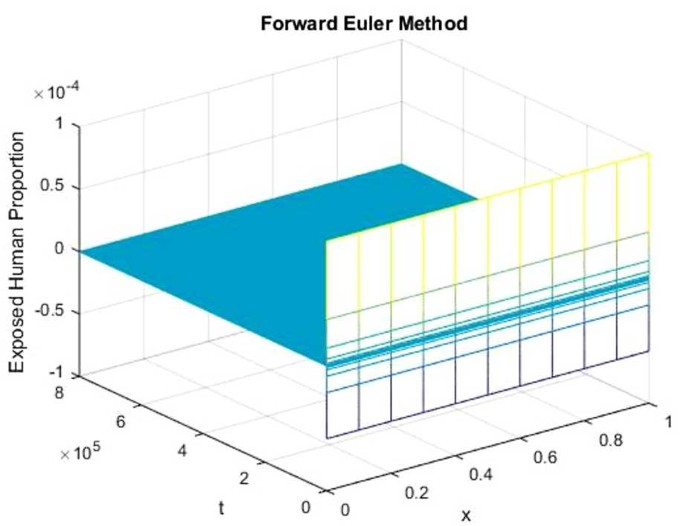Figure 1Figure 2

Figures 12 represent the graphs of exposed population by using the forward Euler and Crank–Nicolson methods. The graphs clearly show that both methods fail to retain positive solution, which is the main feature of the continuous model as we deal with the population dynamics. Now we present the graphs of DFE using the proposed implicit scheme in Figs. 37.Figure 3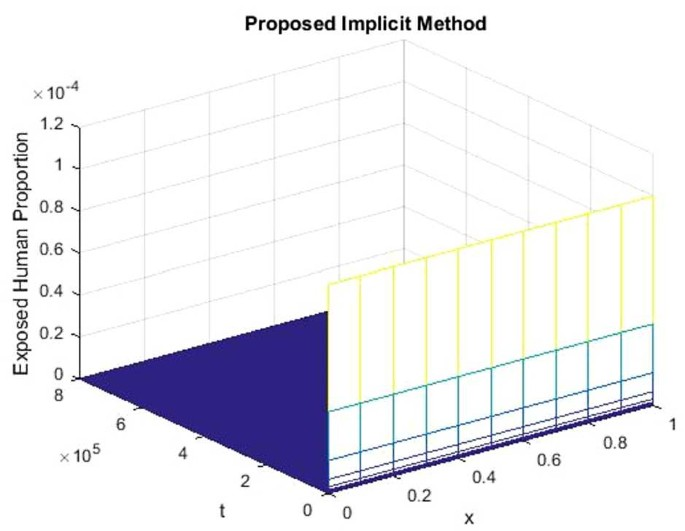Figure 4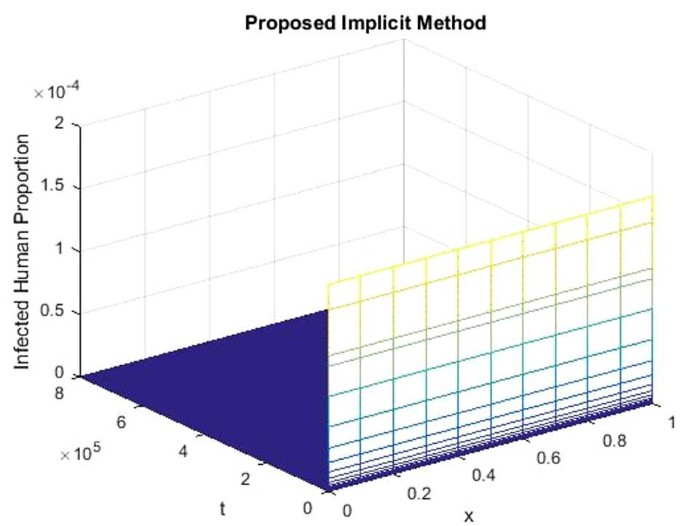Figure 5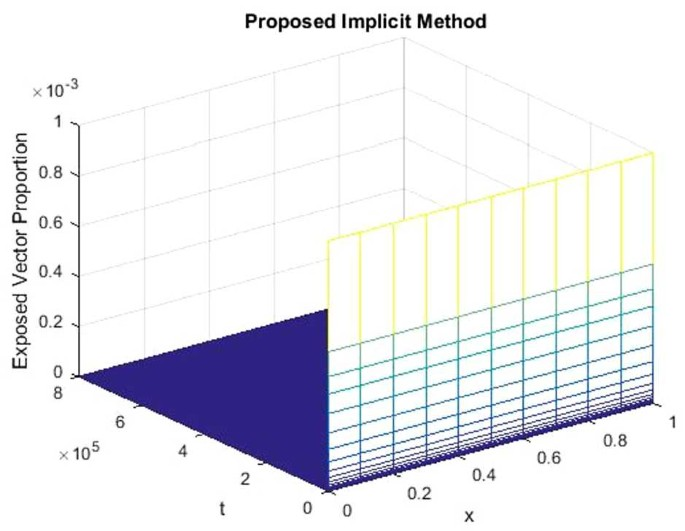Figure 6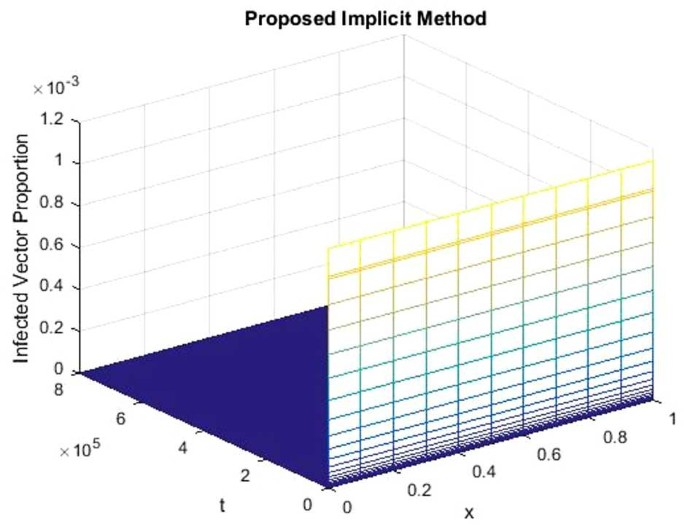Figure 7

Figures 37 clearly show that the proposed implicit scheme converges to disease-free equilibrium point $$E_{0} (1, 0, 0,0,0)$$ and preserves the positivity property.

Next the simulations by using the proposed explicit positive scheme are presented at same equilibrium point as above.

Figures 812 represent the graphs using the proposed FD scheme. These graphs show the disease-free equilibrium. Graphs clearly show that the proposed FD scheme converges to the disease-free equilibrium point $${E}_{{0}} ({1}, {0}, {0},{0},{0})$$ and preserves the positivity property.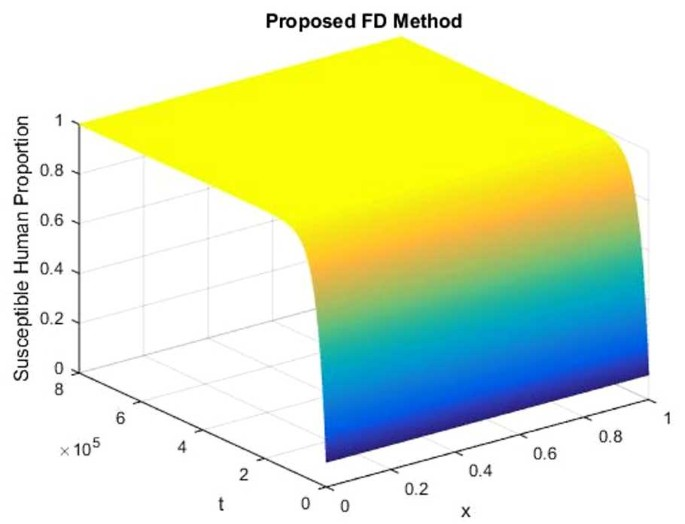Figure 8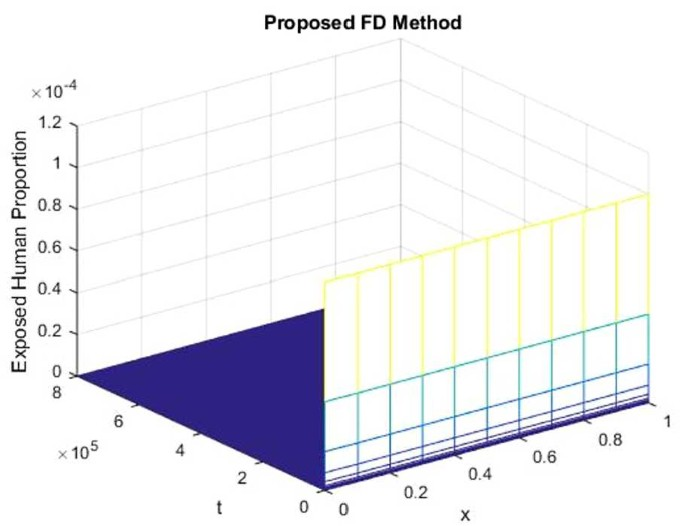Figure 9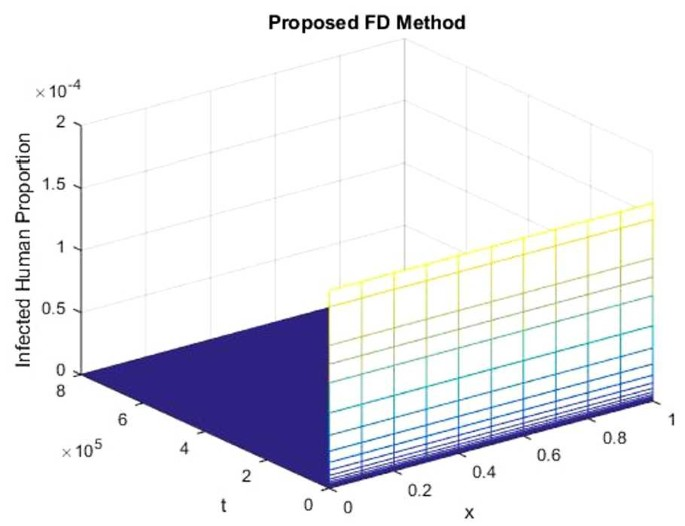Figure 10Figure 11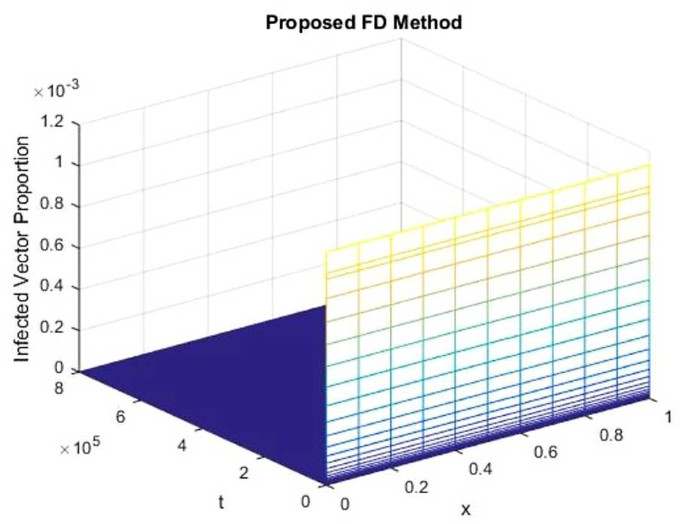Figure 12

#### Endemic equilibrium

The graphs of EE (endemic equilibrium) are presented using four finite difference schemes: forward Euler FD scheme, Crank–Nicolson FD scheme, the proposed implicit scheme and the proposed positive explicit FD scheme.

For the endemic equilibrium, we use $$C=300$$ ($$\mathfrak{R}_{0} >1$$).

Figures 1314 represent the graphical behavior of susceptible individuals at EE for the forward Euler and Crank–Nicolson methods. Graphs clearly show that both schemes demonstrate nonphysical behavior and do not converge to the EE.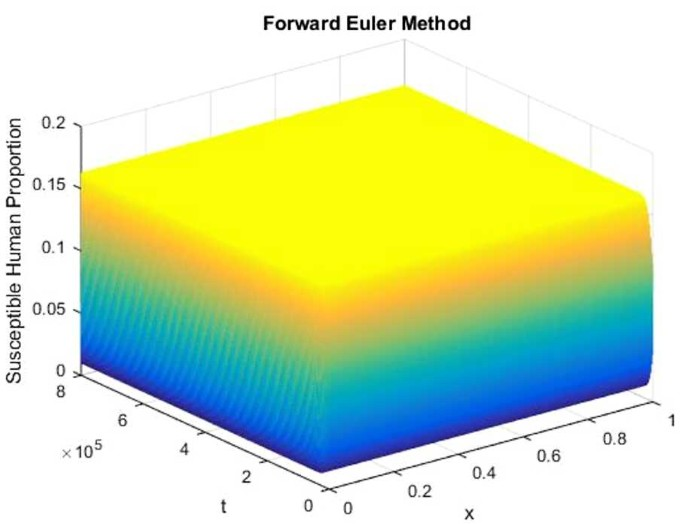Figure 13Figure 14

Figures 1524 represent the graphs of the endemic point by implementing the proposed implicit numerical method and the proposed FD scheme. Graphs clearly show that the proposed schemes converge to the endemic equilibrium point $$E_{1} ( S^{*}, X^{*}, I^{*}, X_{v}^{*}, I_{v}^{*} )$$ and sustain the positive solution.Figure 15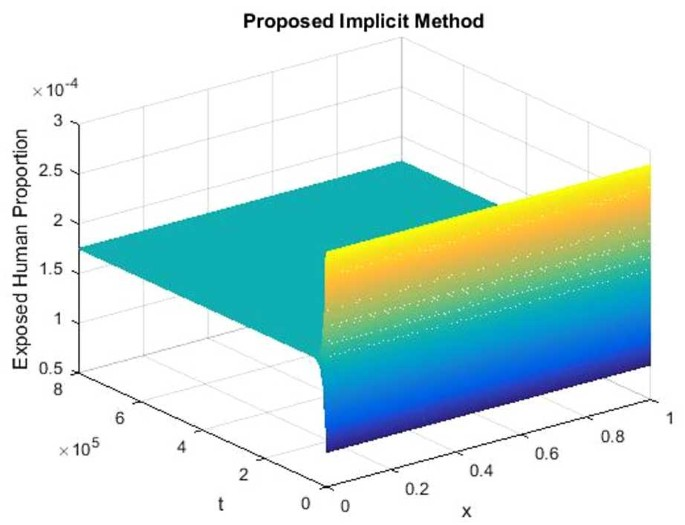Figure 16Figure 17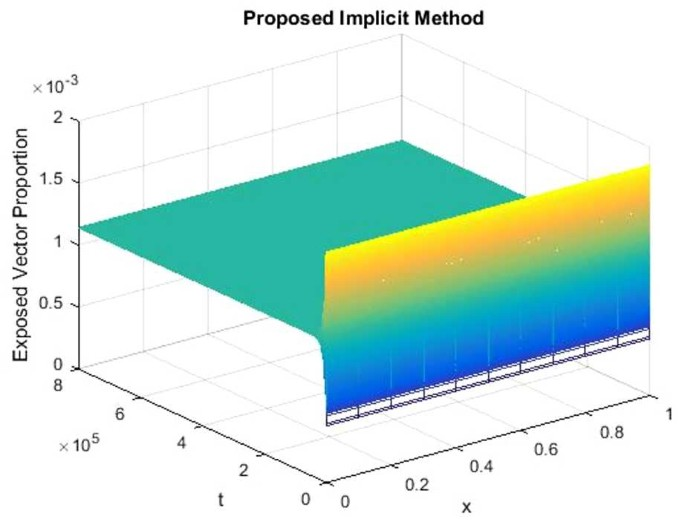Figure 18Figure 19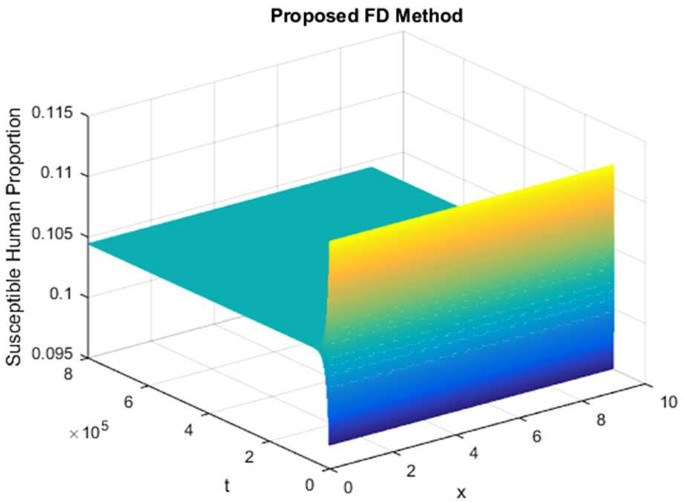Figure 20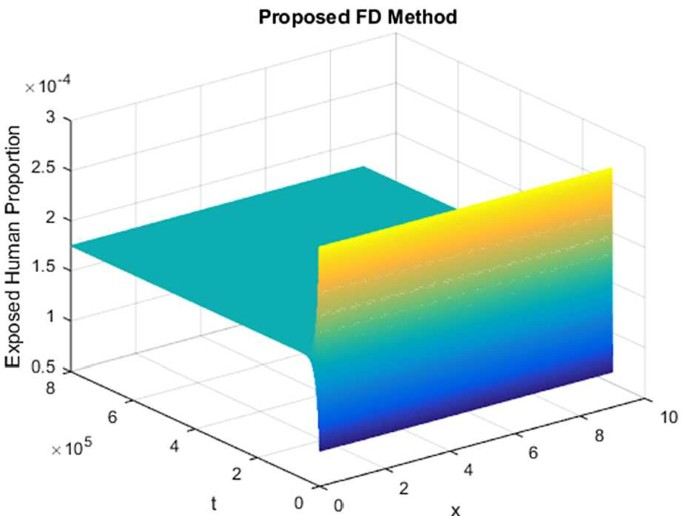Figure 21Figure 22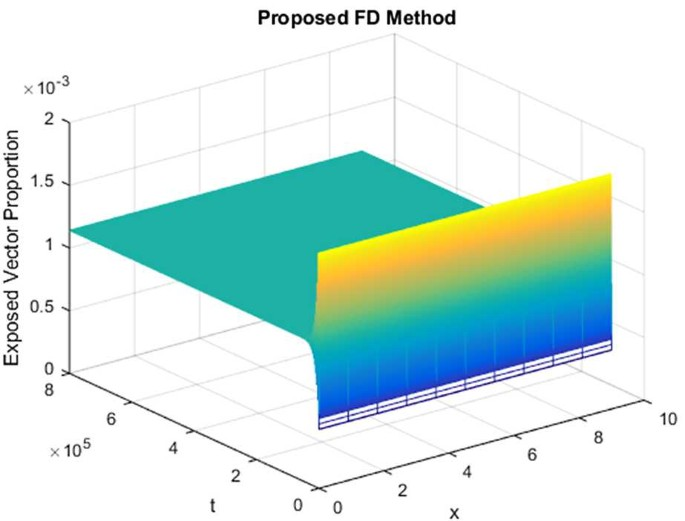Figure 23Figure 24

Now we present the time efficiency at disease-free equilibrium point which is discussed in Table 2.

Table 2 demonstrates that the positive explicit method is time efficient as compared to the positive implicit method. The implicit method takes more than double execution time than the explicit method. This efficiency is shown in the reaction–diffusion model in one space dimension. As far as the complicated situation of two and three space dimensions is concerned, the implication of the proposed implicit scheme is very difficult. We have to observe the long-term behaviour of such a model, therefore time efficiency is crucial for the numerical scheme in a multidimensional space; for the details, see .

## Conclusion

In this paper, we proposed two positive FD schemes to solve a reaction–diffusion dengue epidemic model with incubation period of the virus. We used four FD schemes to solve numerically the reaction–diffusion dengue epidemic model. These schemes were the forward Euler FD scheme, Crank–Nicolson scheme, the proposed implicit FD and the proposed explicit FD schemes. Both existing schemes fail to preserve the positivity property, show nonphysical behavior and converge to false steady states, whereas the proposed FD schemes converge towards the true steady states of the continuous model. The proposed FD schemes are unconditionally dynamically consistent with the positivity property, which is necessary as negative values of a subpopulation are meaningless. Simulations of a test problem were presented in this paper. These simulations show that the proposed implicit and explicit FD schemes converge to all the steady states of the system and preserve the positivity property. The proposed explicit method is time efficient as compared to the implicit method. In the future the proposed explicit scheme will be an important tool to solve many other infectious disease reaction–diffusion mathematical models in multiple space dimensions because of its time efficiency. Our future plans include spatio-temporal numerical analysis of a stochastic dengue epidemic model  and fractional order dynamical systems [32, 33].

## References

1. 1.

Pongsumpun, P.: Mathematical model of dengue disease with incubation period of virus. World Academy of Science, Engineering and Technology 44 (2008)

2. 2.

Naowarat, S., Rajabhat, S.: Dynamical model for determining human susceptibility to dengue fever. Am. J. Appl. Sci. 8(11), 1101–1106 (2011)

3. 3.

Coutinho, F.A.B., Burattini, M.N., Lopez, L.F., Massad, E.: Threshold conditions for a non-autonomous epidemic system describing the population dynamics of dengue. Bull. Math. Biol. 68(8), 2263–2282 (2006)

4. 4.

Favier, C., Degallier, N., Dubois, M.A., Boulanger, J.C., Menkes, C.E., Torres, L., et al.: Dengue epidemic modeling: stakes and pitfalls. Asia-Pac. Biotech News 9(22), 1191–1194 (2005)

5. 5.

Derouich, M., Boutayeb, A., Twizell, E.H.: A model of dengue fever. pp. 1–10, Brunel University, England (2003)

6. 6.

Derouich, M., Boutayeb, A.: Dengue fever: mathematical modelling and computer simulation. Appl. Math. Comput. 177(2), 528–544 (2006)

7. 7.

Syafruddin, S., Noorani, S.M.: SEIR model for transmission of dengue fever in Selangor Malaysia. Int. J. Mod. Phys. Conf. Ser. 9, 380–389 (2012)

8. 8.

Baleanu, D., Rezapour, S., Saberpour, Z.: On fractional integro-differential inclusions via the extended fractional Caputo–Fabrizio derivation. Bound. Value Probl. 2019, 79 (2019)

9. 9.

Baleanu, D., Ghafarnezhad, K., Rezapour, S., Shabibi, M.: On the existence of solutions of a three steps crisis integro-differential equation. Adv. Differ. Equ. 2018, 135 (2018)

10. 10.

Baleanu, D., Etemad, S., Pourrazi, S., Rezapour, Sh.: On the new fractional hybrid boundary value problems with three-point integral hybrid conditions. Adv. Differ. Equ. 2019, 473 (2019)

11. 11.

Rafiq, M., Ahmad, M.O.: Numerical modeling of dengue disease with incubation period of virus. Pak. J. Eng. Appl. Sci. 17, 19–29 (2015)

12. 12.

Mickens, R.E.: Nonstandard Finite Difference Models of Differential Equations. World Scientific, Singapore (1994)

13. 13.

Rafiq, M., Raza, A., Rafia: Numerical modeling of transmission dynamics of vector-borne plant pathogen. In: 2017 14th International Bhurban Conference on Applied Sciences and Technology (IBCAST), Islamabad, pp. 214–219 (2017)

14. 14.

Jansen, H., Twizell, E.H.: An unconditionally convergent discretization of the SEIR model. Math. Comput. Simul. 58, 147–158 (2002)

15. 15.

Rafiq, M., Ahmad, M.O., Iqbal, S.: Numerical modeling of internal transmission dynamics of dengue virus. In: 2016 13th International Bhurban Conference on Applied Sciences and Technology (IBCAST), Islamabad, pp. 85–91 (2016)

16. 16.

Jódor, L., Villanueva, R.J., Arenas, A.J., González, G.: Nonstandard numerical methods for a mathematical model for influenza disease. Comput. Math. Appl. 79, 622–633 (2008)

17. 17.

Arenas, A.J., Parra, G.G., Chen-Charpentier, B.M.: A nonstandard numerical scheme of predictor–corrector type for epidemic models. Comput. Math. Appl. 59(12), 3740–3749 (2010)

18. 18.

Piawong, W., Twizell, E.H., Gumel, A.B.: An unconditionally convergent finite difference scheme for the SIR model. Appl. Math. Comput. 146, 611–625 (2003)

19. 19.

Chapwanya, M., Lubuma, J.M.-S., Mickens, R.E.: Positivity-preserving nonstandard finite difference schemes for cross-diffusion equations in biosciences. Comput. Math. Appl. 68(9), 1071–1082 (2014)

20. 20.

Fatima, U., Ali, M., Ahmed, N., Rafiq, M.: Numerical modeling of susceptible latent breaking-out quarantine computer virus epidemic dynamics. Heliyon 4, e00631 (2018)

21. 21.

Ahmed, N., Jawaz, M., Rafiq, M., Rehman, M.A., Ali, M., Ahmad, M.O.: Numerical treatment of an epidemic model with spatial diffusion. J. Appl. Environ. Biol. Sci. 8(6), 17–29 (2018)

22. 22.

Ahmed, N., Shahid, N., Iqbal, Z., Jawaz, M., Rafiq, M., Tahira, S.S., Ahmad, M.O.: Numerical modeling of SEIQV epidemic model with saturated incidence rate. J. Appl. Environ. Biol. Sci. 8(4), 67–82 (2018)

23. 23.

Chinviriyasit, S., Chinviriyasit, W.: Numerical modeling of SIR epidemic model with diffusion. Appl. Math. Comput. 216, 395–409 (2010)

24. 24.

Jaichuang, A., Chinviriyasit, W.: Numerical modelling of influenza model with diffusion. Int. J. Appl. Phys. Math. 4(1), 15–21 (2014)

25. 25.

Somjaivang, D., Chinviriyasit, S.: Numerical modeling of an influenza epidemic model with vaccination and diffusion. Int. J. Appl. Phys. Math. 4(1), 68–74 (2014)

26. 26.

Qin, W., Wang, L., Ding, X.: A non-standard finite difference method for a hepatitis B virus infection model with spatial diffusion. J. Differ. Equ. Appl. 20(12), 1641–1651 (2014)

27. 27.

Appadu, A.R., Lubuma, J.M.-S., Mphephu, N.: Computation study of three numerical methods for some linear and nonlinear advection–diffusion–reaction problems. Prog. Comput. Fluid Dyn. 17, 114–129 (2017)

28. 28.

Chen-Charpentier, B.M., Kojouharov, H.V.: Unconditionally positivity preserving scheme for advection–diffusion–reaction equations. Math. Comput. Model. 57, 2177–2185 (2013)

29. 29.

Ahmed, N., Rafiq, M., Rehman, M.A., Iqbal, M.S., Ali, M.: Numerical modelling of three dimensional Brusselator reaction diffusion system. AIP Adv. 9, 015205 (2019)

30. 30.

Ahmed, N., Rafiq, M., Rehman, M.A., Ali, M., Ahmad, M.O.: Numerical modeling of SEIR measles dynamics with diffusion. Commun. Math. Appl. 9(3), 315–326 (2018)

31. 31.

Raza, A., Arif, M.S., Rafiq, M.: A reliable numerical analysis for stochastic dengue epidemic model with incubation period of virus. Adv. Differ. Equ. 2019, 32 (2019)

32. 32.

Baleanu, D., Jajarmi, A., Bonyah, E., Hajipour, M.: New aspects of poor nutrition in the life cycle within the fractional calculus. Adv. Differ. Equ. 2018, 230 (2018)

33. 33.

Baleanu, D., Doha, E.H., Abdelkawy, M.A., Amin, A.Z.M.: Approximate solutions for solving nonlinear variable-order fractional Riccati differential equations. Nonlinear Anal. Model. Control 24, 176–188 (2019)

Download references

### Acknowledgements

We are thankful to the reviewers for their valuable suggestions and comments to enhance the quality of this research work.

Not applicable.

No funding.

## Author information

Authors

### Contributions

All the authors have contributed equally. All authors read and approved the final manuscript.

### Corresponding author

Correspondence to Dumitru Baleanu.

## Ethics declarations

### Competing interests

The authors declare that they have no competing interests.

## Rights and permissions

Open Access This article is licensed under a Creative Commons Attribution 4.0 International License, which permits use, sharing, adaptation, distribution and reproduction in any medium or format, as long as you give appropriate credit to the original author(s) and the source, provide a link to the Creative Commons licence, and indicate if changes were made. The images or other third party material in this article are included in the article’s Creative Commons licence, unless indicated otherwise in a credit line to the material. If material is not included in the article’s Creative Commons licence and your intended use is not permitted by statutory regulation or exceeds the permitted use, you will need to obtain permission directly from the copyright holder. To view a copy of this licence, visit http://creativecommons.org/licenses/by/4.0/.

Reprints and Permissions